Calculus Volume 1

# 6.3Volumes of Revolution: Cylindrical Shells

Calculus Volume 16.3 Volumes of Revolution: Cylindrical Shells

### Learning Objectives

• 6.3.1 Calculate the volume of a solid of revolution by using the method of cylindrical shells.
• 6.3.2 Compare the different methods for calculating a volume of revolution.

In this section, we examine the method of cylindrical shells, the final method for finding the volume of a solid of revolution. We can use this method on the same kinds of solids as the disk method or the washer method; however, with the disk and washer methods, we integrate along the coordinate axis parallel to the axis of revolution. With the method of cylindrical shells, we integrate along the coordinate axis perpendicular to the axis of revolution. The ability to choose which variable of integration we want to use can be a significant advantage with more complicated functions. Also, the specific geometry of the solid sometimes makes the method of using cylindrical shells more appealing than using the washer method. In the last part of this section, we review all the methods for finding volume that we have studied and lay out some guidelines to help you determine which method to use in a given situation.

### The Method of Cylindrical Shells

Again, we are working with a solid of revolution. As before, we define a region $R,R,$ bounded above by the graph of a function $y=f(x),y=f(x),$ below by the $x-axis,x-axis,$ and on the left and right by the lines $x=ax=a$ and $x=b,x=b,$ respectively, as shown in Figure 6.25(a). We then revolve this region around the y-axis, as shown in Figure 6.25(b). Note that this is different from what we have done before. Previously, regions defined in terms of functions of $xx$ were revolved around the $x-axisx-axis$ or a line parallel to it.

Figure 6.25 (a) A region bounded by the graph of a function of $x.x.$ (b) The solid of revolution formed when the region is revolved around the $y-axis.y-axis.$

As we have done many times before, partition the interval $[a,b][a,b]$ using a regular partition, $P={x0,x1,…,xn}P={x0,x1,…,xn}$ and, for $i=1,2,…,n,i=1,2,…,n,$ choose a point $xi*∈[xi−1,xi].xi*∈[xi−1,xi].$ Then, construct a rectangle over the interval $[xi−1,xi][xi−1,xi]$ of height $f(xi*)f(xi*)$ and width $Δx.Δx.$ A representative rectangle is shown in Figure 6.26(a). When that rectangle is revolved around the y-axis, instead of a disk or a washer, we get a cylindrical shell, as shown in the following figure.

Figure 6.26 (a) A representative rectangle. (b) When this rectangle is revolved around the $y-axis,y-axis,$ the result is a cylindrical shell. (c) When we put all the shells together, we get an approximation of the original solid.

To calculate the volume of this shell, consider Figure 6.27.

Figure 6.27 Calculating the volume of the shell.

The shell is a cylinder, so its volume is the cross-sectional area multiplied by the height of the cylinder. The cross-sections are annuli (ring-shaped regions—essentially, circles with a hole in the center), with outer radius $xixi$ and inner radius $xi−1.xi−1.$ Thus, the cross-sectional area is $πxi2−πxi−12.πxi2−πxi−12.$ The height of the cylinder is $f(xi*).f(xi*).$ Then the volume of the shell is

$Vshell=f(xi*)(πxi2−πxi−12)=πf(xi*)(xi2−xi−12)=πf(xi*)(xi+xi−1)(xi−xi−1)=2πf(xi*)(xi+xi−12)(xi−xi−1).Vshell=f(xi*)(πxi2−πxi−12)=πf(xi*)(xi2−xi−12)=πf(xi*)(xi+xi−1)(xi−xi−1)=2πf(xi*)(xi+xi−12)(xi−xi−1).$

Note that $xi−xi−1=Δx,xi−xi−1=Δx,$ so we have

$Vshell=2πf(xi*)(xi+xi−12)Δx.Vshell=2πf(xi*)(xi+xi−12)Δx.$

Furthermore, $xi+xi−12xi+xi−12$ is both the midpoint of the interval $[xi−1,xi][xi−1,xi]$ and the average radius of the shell, and we can approximate this by $xi*.xi*.$ We then have

$Vshell≈2πf(xi*)xi*Δx.Vshell≈2πf(xi*)xi*Δx.$

Another way to think of this is to think of making a vertical cut in the shell and then opening it up to form a flat plate (Figure 6.28).

Figure 6.28 (a) Make a vertical cut in a representative shell. (b) Open the shell up to form a flat plate.

In reality, the outer radius of the shell is greater than the inner radius, and hence the back edge of the plate would be slightly longer than the front edge of the plate. However, we can approximate the flattened shell by a flat plate of height $f(xi*),f(xi*),$ width $2πxi*,2πxi*,$ and thickness $ΔxΔx$ (Figure 6.28). The volume of the shell, then, is approximately the volume of the flat plate. Multiplying the height, width, and depth of the plate, we get

$Vshell≈f(xi*)(2πxi*)Δx,Vshell≈f(xi*)(2πxi*)Δx,$

which is the same formula we had before.

To calculate the volume of the entire solid, we then add the volumes of all the shells and obtain

$V≈∑i=1n(2πxi*f(xi*)Δx).V≈∑i=1n(2πxi*f(xi*)Δx).$

Here we have another Riemann sum, this time for the function $2πxf(x).2πxf(x).$ Taking the limit as $n→∞n→∞$ gives us

$V=limn→∞∑i=1n(2πxi*f(xi*)Δx)=∫ab(2πxf(x))dx.V=limn→∞∑i=1n(2πxi*f(xi*)Δx)=∫ab(2πxf(x))dx.$

This leads to the following rule for the method of cylindrical shells.

### Rule: The Method of Cylindrical Shells

Let $f(x)f(x)$ be continuous and nonnegative. Define $RR$ as the region bounded above by the graph of $f(x),f(x),$ below by the $x-axis,x-axis,$ on the left by the line $x=a,x=a,$ and on the right by the line $x=b.x=b.$ Then the volume of the solid of revolution formed by revolving $RR$ around the y-axis is given by

$V=∫ab(2πxf(x))dx.V=∫ab(2πxf(x))dx.$
(6.6)

Now let’s consider an example.

### Example 6.12

#### The Method of Cylindrical Shells 1

Define $RR$ as the region bounded above by the graph of $f(x)=1/xf(x)=1/x$ and below by the $x-axisx-axis$ over the interval $[1,3].[1,3].$ Find the volume of the solid of revolution formed by revolving $RR$ around the $y-axis.y-axis.$

### Checkpoint6.12

Define R as the region bounded above by the graph of $f(x)=x2f(x)=x2$ and below by the x-axis over the interval $[1,2].[1,2].$ Find the volume of the solid of revolution formed by revolving $RR$ around the $y-axis.y-axis.$

### Example 6.13

#### The Method of Cylindrical Shells 2

Define R as the region bounded above by the graph of $f(x)=2x−x2f(x)=2x−x2$ and below by the $x-axisx-axis$ over the interval $[0,2].[0,2].$ Find the volume of the solid of revolution formed by revolving $RR$ around the $y-axis.y-axis.$

### Checkpoint6.13

Define $RR$ as the region bounded above by the graph of $f(x)=3x−x2f(x)=3x−x2$ and below by the $x-axisx-axis$ over the interval $[0,2].[0,2].$ Find the volume of the solid of revolution formed by revolving $RR$ around the $y-axis.y-axis.$

As with the disk method and the washer method, we can use the method of cylindrical shells with solids of revolution, revolved around the $x-axis,x-axis,$ when we want to integrate with respect to $y.y.$ The analogous rule for this type of solid is given here.

### Rule: The Method of Cylindrical Shells for Solids of Revolution around the x-axis

Let $g(y)g(y)$ be continuous and nonnegative. Define $QQ$ as the region bounded on the right by the graph of $g(y),g(y),$ on the left by the $y-axis,y-axis,$ below by the line $y=c,y=c,$ and above by the line $y=d.y=d.$ Then, the volume of the solid of revolution formed by revolving $QQ$ around the $x-axisx-axis$ is given by

$V=∫cd(2πyg(y))dy.V=∫cd(2πyg(y))dy.$

### Example 6.14

#### The Method of Cylindrical Shells for a Solid Revolved around the x-axis

Define $QQ$ as the region bounded on the right by the graph of $g(y)=2yg(y)=2y$ and on the left by the $y-axisy-axis$ for $y∈[0,4].y∈[0,4].$ Find the volume of the solid of revolution formed by revolving $QQ$ around the x-axis.

### Checkpoint6.14

Define $QQ$ as the region bounded on the right by the graph of $g(y)=3/yg(y)=3/y$ and on the left by the $y-axisy-axis$ for $y∈[1,3].y∈[1,3].$ Find the volume of the solid of revolution formed by revolving $QQ$ around the $x-axis.x-axis.$

For the next example, we look at a solid of revolution for which the graph of a function is revolved around a line other than one of the two coordinate axes. To set this up, we need to revisit the development of the method of cylindrical shells. Recall that we found the volume of one of the shells to be given by

$Vshell=f(xi*)(πxi2−πxi−12)=πf(xi*)(xi2−xi−12)=πf(xi*)(xi+xi−1)(xi−xi−1)=2πf(xi*)(xi+xi−12)(xi−xi−1).Vshell=f(xi*)(πxi2−πxi−12)=πf(xi*)(xi2−xi−12)=πf(xi*)(xi+xi−1)(xi−xi−1)=2πf(xi*)(xi+xi−12)(xi−xi−1).$

This was based on a shell with an outer radius of $xixi$ and an inner radius of $xi−1.xi−1.$ If, however, we rotate the region around a line other than the $y-axis,y-axis,$ we have a different outer and inner radius. Suppose, for example, that we rotate the region around the line $x=−k,x=−k,$ where $kk$ is some positive constant. Then, the outer radius of the shell is $xi+kxi+k$ and the inner radius of the shell is $xi−1+k.xi−1+k.$ Substituting these terms into the expression for volume, we see that when a plane region is rotated around the line $x=−k,x=−k,$ the volume of a shell is given by

$Vshell=2πf(xi*)((xi+k)+(xi−1+k)2)((xi+k)−(xi−1+k))=2πf(xi*)((xi+xi−22)+k)Δx.Vshell=2πf(xi*)((xi+k)+(xi−1+k)2)((xi+k)−(xi−1+k))=2πf(xi*)((xi+xi−22)+k)Δx.$

As before, we notice that $xi+xi−12xi+xi−12$ is the midpoint of the interval $[xi−1,xi][xi−1,xi]$ and can be approximated by $xi*.xi*.$ Then, the approximate volume of the shell is

$Vshell≈2π(xi*+k)f(xi*)Δx.Vshell≈2π(xi*+k)f(xi*)Δx.$

The remainder of the development proceeds as before, and we see that

$V=∫ab(2π(x+k)f(x))dx.V=∫ab(2π(x+k)f(x))dx.$

We could also rotate the region around other horizontal or vertical lines, such as a vertical line in the right half plane. In each case, the volume formula must be adjusted accordingly. Specifically, the $x-termx-term$ in the integral must be replaced with an expression representing the radius of a shell. To see how this works, consider the following example.

### Example 6.15

#### A Region of Revolution Revolved around a Line

Define $RR$ as the region bounded above by the graph of $f(x)=xf(x)=x$ and below by the $x-axisx-axis$ over the interval $[1,2].[1,2].$ Find the volume of the solid of revolution formed by revolving $RR$ around the line $x=−1.x=−1.$

### Checkpoint6.15

Define $RR$ as the region bounded above by the graph of $f(x)=x2f(x)=x2$ and below by the $x-axisx-axis$ over the interval $[0,1].[0,1].$ Find the volume of the solid of revolution formed by revolving $RR$ around the line $x=−2.x=−2.$

For our final example in this section, let’s look at the volume of a solid of revolution for which the region of revolution is bounded by the graphs of two functions.

### Example 6.16

#### A Region of Revolution Bounded by the Graphs of Two Functions

Define $RR$ as the region bounded above by the graph of the function $f(x)=xf(x)=x$ and below by the graph of the function $g(x)=1/xg(x)=1/x$ over the interval $[1,4].[1,4].$ Find the volume of the solid of revolution generated by revolving $RR$ around the $y-axis.y-axis.$

### Checkpoint6.16

Define $RR$ as the region bounded above by the graph of $f(x)=xf(x)=x$ and below by the graph of $g(x)=x2g(x)=x2$ over the interval $[0,1].[0,1].$ Find the volume of the solid of revolution formed by revolving $RR$ around the $y-axis.y-axis.$

### Which Method Should We Use?

We have studied several methods for finding the volume of a solid of revolution, but how do we know which method to use? It often comes down to a choice of which integral is easiest to evaluate. Figure 6.34 describes the different approaches for solids of revolution around the $x-axis.x-axis.$ It’s up to you to develop the analogous table for solids of revolution around the $y-axis.y-axis.$

Figure 6.34

Let’s take a look at a couple of additional problems and decide on the best approach to take for solving them.

### Example 6.17

#### Selecting the Best Method

For each of the following problems, select the best method to find the volume of a solid of revolution generated by revolving the given region around the $x-axis,x-axis,$ and set up the integral to find the volume (do not evaluate the integral).

1. The region bounded by the graphs of $y=x,y=x,$ $y=2−x,y=2−x,$ and the $x-axis.x-axis.$
2. The region bounded by the graphs of $y=4x−x2y=4x−x2$ and the $x-axis.x-axis.$

### Checkpoint6.17

Select the best method to find the volume of a solid of revolution generated by revolving the given region around the $x-axis,x-axis,$ and set up the integral to find the volume (do not evaluate the integral): the region bounded by the graphs of $y=2−x2y=2−x2$ and $y=x2.y=x2.$

### Section 6.3 Exercises

For the following exercises, find the volume generated when the region between the two curves is rotated around the given axis. Use both the shell method and the washer method. Use technology to graph the functions and draw a typical slice by hand.

114.

[T] Bounded by the curves $y=3x,x=0,y=3x,x=0,$ and $y=3y=3$ rotated around the $y-axis.y-axis.$

115.

[T] Bounded by the curves $y=3x,y=0,andx=3y=3x,y=0,andx=3$ rotated around the $y-axis.y-axis.$

116.

[T] Bounded by the curves $y=3x,y=0,andy=3y=3x,y=0,andy=3$ rotated around the $x-axis.x-axis.$

117.

[T] Bounded by the curves $y=3x,y=0,andx=3y=3x,y=0,andx=3$ rotated around the $x-axis.x-axis.$

118.

[T] Bounded by the curves $y=2x3,y=0,andx=2y=2x3,y=0,andx=2$ rotated around the $y-axis.y-axis.$

119.

[T] Bounded by the curves $y=2x3,y=0,andx=2y=2x3,y=0,andx=2$ rotated around the $x-axis.x-axis.$

For the following exercises, use shells to find the volumes of the given solids. Note that the rotated regions lie between the curve and the $x-axisx-axis$ and are rotated around the $y-axis.y-axis.$

120.

$y = 1 − x 2 , x = 0 , and x = 1 y = 1 − x 2 , x = 0 , and x = 1$

121.

$y = 5 x 3 , x = 0 , and x = 1 y = 5 x 3 , x = 0 , and x = 1$

122.

$y = 1 x , x = 1 , and x = 100 y = 1 x , x = 1 , and x = 100$

123.

$y = 1 − x 2 , x = 0 , and x = 1 y = 1 − x 2 , x = 0 , and x = 1$

124.

$y = 1 1 + x 2 , x = 0 , and x = 3 y = 1 1 + x 2 , x = 0 , and x = 3$

125.

$y = sin x 2 , x = 0 , and x = π y = sin x 2 , x = 0 , and x = π$

126.

$y = 1 1 − x 2 , x = 0 , and x = 1 2 y = 1 1 − x 2 , x = 0 , and x = 1 2$

127.

$y = x , x = 0 , and x = 1 y = x , x = 0 , and x = 1$

128.

$y = ( 1 + x 2 ) 3 , x = 0 , and x = 1 y = ( 1 + x 2 ) 3 , x = 0 , and x = 1$

129.

$y = 5 x 3 − 2 x 4 , x = 0 , and x = 2 y = 5 x 3 − 2 x 4 , x = 0 , and x = 2$

For the following exercises, use shells to find the volume generated by rotating the regions between the given curve and $y=0y=0$ around the $x-axis.x-axis.$

130.

$y=1−x2,x=0,x=1y=1−x2,x=0,x=1$ and the x-axis

131.

$y=x2,x=0,x=2y=x2,x=0,x=2$ and the x-axis

132.

$y=x32,x=0,x=2,y=x32,x=0,x=2,$ and the x-axis

133.

$y=2x2,x=1,x=2,y=2x2,x=1,x=2,$ and the x-axis

134.

$x = 1 1 + y 2 , y = 1 , and y = 4 x = 1 1 + y 2 , y = 1 , and y = 4$

135.

$x=1+y2y,y=1,y=4,x=1+y2y,y=1,y=4,$ and the y-axis

136.

$x=cosy,x=0,andy=π x = cos y , x = 0 , and y = π$

137.

$x=y3-2y2,x=0,x=9x=y3-2y2,x=0,x=9$

138.

$x=y+1,x=1,x=3,x=y+1,x=1,x=3,$ and the x-axis

139.

$x = 27 y 3 and x = 3 y 4 x = 27 y 3 and x = 3 y 4$

For the following exercises, find the volume generated when the region between the curves is rotated around the given axis.

140.

$y=3−x,y=0,x=0,andx=2y=3−x,y=0,x=0,andx=2$ rotated around the $y-axis.y-axis.$

141.

$y=x3,x=0,andy=8y=x3,x=0,andy=8$ rotated around the $y-axis.y-axis.$

142.

$y=x2,y=x,y=x2,y=x,$ rotated around the $y-axis.y-axis.$

143.

$y=x,y=0,andx=1y=x,y=0,andx=1$ rotated around the line $x=2.x=2.$

144.

$y=14−x,x=1,x=2andy=0y=14−x,x=1,x=2andy=0$ rotated around the line $x=4.x=4.$

145.

$y=xandy=x2y=xandy=x2$ rotated around the $y-axis.y-axis.$

146.

$y=xandy=x2y=xandy=x2$ rotated around the line $x=2.x=2.$

147.

$x=y3,x=1y,x=1,andx=2x=y3,x=1y,x=1,andx=2$ rotated around the $x-axis.x-axis.$

148.

$x=y2andy=xx=y2andy=x$ rotated around the line $y=2.y=2.$

149.

[T] Left of $x=sin(πy),x=sin(πy),$ right of $y=x,y=x,$ around the $y-axis.y-axis.$

For the following exercises, use technology to graph the region. Determine which method you think would be easiest to use to calculate the volume generated when the function is rotated around the specified axis. Then, use your chosen method to find the volume.

150.

[T] $y=x2y=x2$ and $y=4xy=4x$ rotated around the $y-axis.y-axis.$

151.

[T] $y=cos(πx),y=sin(πx),x=14,andx=54y=cos(πx),y=sin(πx),x=14,andx=54$ rotated around the $y-axis.y-axis.$ This exercise requires advanced technique. You may use technology to perform the integration.

152.

[T] $y=x2−2x,x=2,andx=4y=x2−2x,x=2,andx=4$ rotated around the $y-axis.y-axis.$

153.

[T] $y=x2−2x,x=2,andx=4y=x2−2x,x=2,andx=4$ rotated around the $x-axis.x-axis.$

154.

[T] $y=3x3−2,y=x,andx=2y=3x3−2,y=x,andx=2$ rotated around the $x-axis.x-axis.$

155.

[T] $y=3x3−2,y=x,andx=2y=3x3−2,y=x,andx=2$ rotated around the $y-axis.y-axis.$

156.

[T] $x=sin(πy2)x=sin(πy2)$ and $x=2yx=2y$ rotated around the $x-axis.x-axis.$

157.

[T] $x=y2,x=y2−2y+1,andx=2x=y2,x=y2−2y+1,andx=2$ rotated around the $y-axis.y-axis.$

For the following exercises, use the method of shells to approximate the volumes of some common objects, which are pictured in accompanying figures.

158.

Use the method of shells to find the volume of a sphere of radius $r.r.$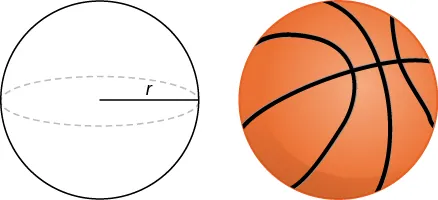159.

Use the method of shells to find the volume of a cone with radius $rr$ and height $h.h.$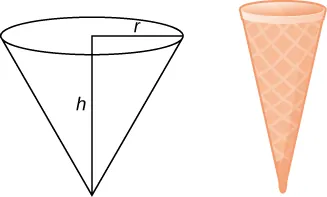160.

Use the method of shells to find the volume of an ellipsoid $(x2/a2)+(y2/b2)=1(x2/a2)+(y2/b2)=1$ rotated around the $x-axis.x-axis.$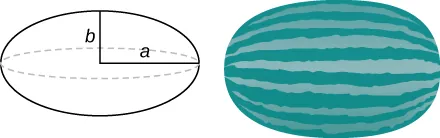161.

Use the method of shells to find the volume of a cylinder with radius $rr$ and height $h.h.$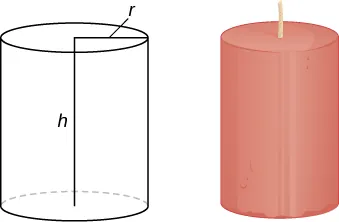162.

Use the method of shells to find the volume of the donut created when the circle $x2+y2=4x2+y2=4$ is rotated around the line $x=4.x=4.$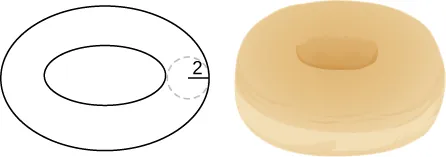163.

Consider he region enclosed by the graphs of $y=f(x),y=1+f(x),x=0,y=0,y=f(x),y=1+f(x),x=0,y=0,$ and $x=a>0.x=a>0.$ What is the volume of the solid generated when this region is rotated around the $y-axis?y-axis?$ Assume that the function is defined over the interval $[0,a].[0,a].$

164.

Consider the function $y=f(x),y=f(x),$ which decreases from $f(0)=bf(0)=b$ to $f(1)=0.f(1)=0.$ Set up the integrals for determining the volume, using both the shell method and the disk method, of the solid generated when this region, with $x=0x=0$ and $y=0,y=0,$ is rotated around the $y-axis.y-axis.$ Prove that both methods approximate the same volume. Which method is easier to apply? (Hint: Since $f(x)f(x)$ is one-to-one, there exists an inverse $f−1(y).)f−1(y).)$

Order a print copy

As an Amazon Associate we earn from qualifying purchases.